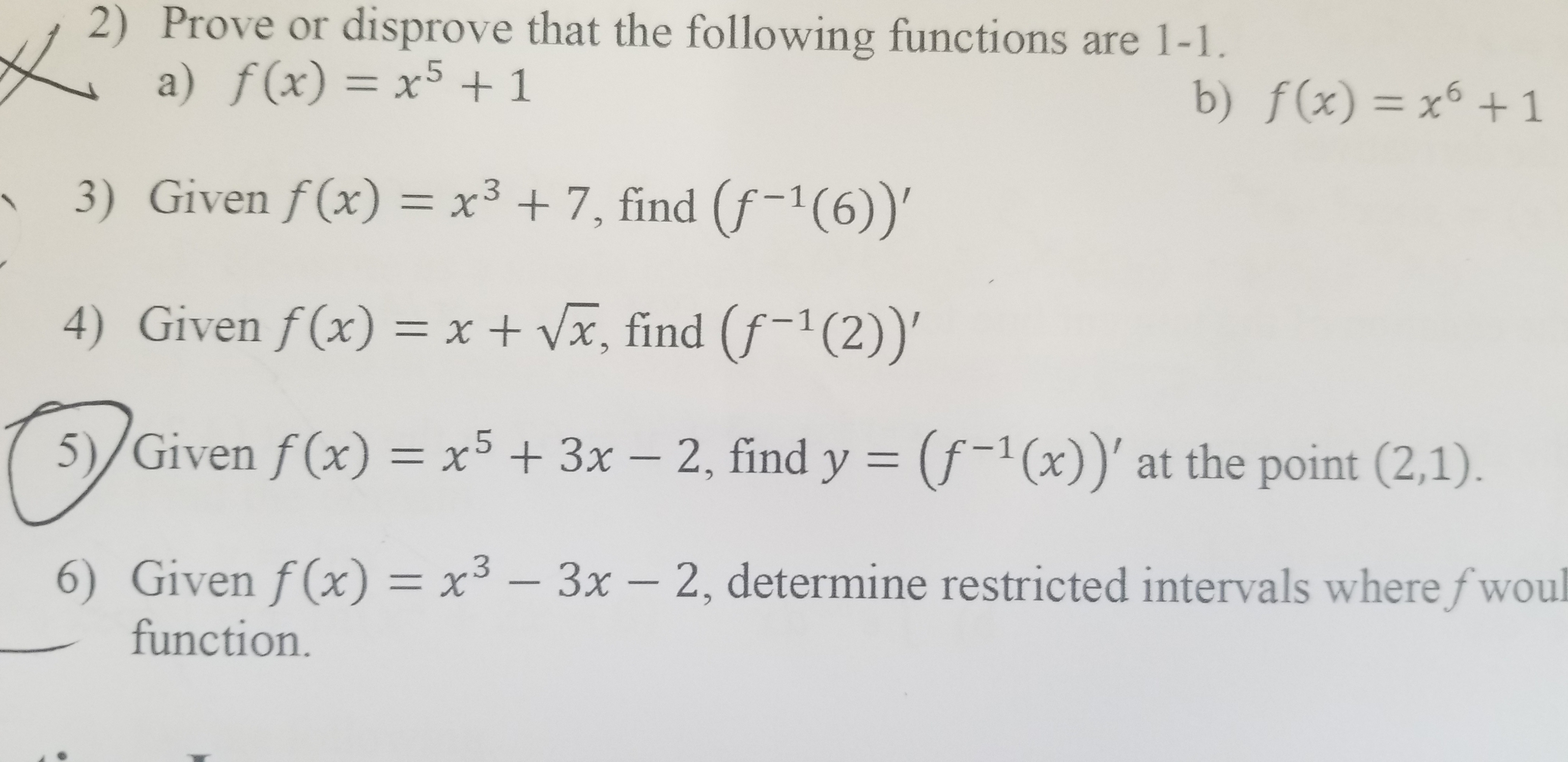# |2) Prove or disprove that the following functions are 1-1a) f(x) = x5 +1b) f(x) = x1-63) Given f(x) = x3 +7, find (f-1(6))'4) Given f(x) = x + Vx, find (f-1 (2))'5)/Given f(x) = x5 + 3x - 2, find y (f-1(x))' at the point (2,1).6) Given f(x) = x3 -3x- 2, determine restricted intervals where fwoulfunction.

Question
20 views

Given f(x) = x^3 + 3x - 2 , find y = (f ^-1 (x))' at the point (2,1)help_outlineImage Transcriptionclose| 2) Prove or disprove that the following functions are 1-1 a) f(x) = x5 +1 b) f(x) = x1 -6 3) Given f(x) = x3 +7, find (f-1(6))' 4) Given f(x) = x + Vx, find (f-1 (2))' 5)/Given f(x) = x5 + 3x - 2, find y (f-1(x))' at the point (2,1). 6) Given f(x) = x3 - 3x- 2, determine restricted intervals where fwoul function. fullscreen
check_circle

Step 1
Step 2

Given information:

The given function is

Step 3

Calculate:

First, Differentiate f(x) with r...

### Want to see the full answer?

See Solution

#### Want to see this answer and more?

Solutions are written by subject experts who are available 24/7. Questions are typically answered within 1 hour.*

See Solution
*Response times may vary by subject and question.
Tagged in

### Derivative